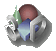# 信息框

##创建新信息框

• Module development页学习如何创建模块。
• 创建信息框文件到 htdocs/mymodule/core/boxes/
• 在模块文件内加入以下代码：
```\$this->boxes = array(
0 => array(
'file' => 'box_myownbox@mymodule',
'note' => 'My notes',
'enabledbydefaulton' => 'Home'
)
// You can declare as much boxes as you want by simply incrementing the index.
);
```
• 为发布，打包包含您的信息框的模块（参见模块开发页）。

## 编辑信息框的内容

### 最简单的HelloWorld

• 首先，先建立一个模块，名为Helloworld，参见模块开发
• 在模块文件内加入以下代码：
```\$this->boxes = array(
0 => array(
'file' => 'helloworldbox@helloworld',
'note' => 'My notes',
'enabledbydefaulton' => 'Home'
)
// You can declare as much boxes as you want by simply incrementing the index.
);
```
• 复制一个信息框文件并重命名为 helloworld\core\boxes\helloworldbox.php
• 修改文件内容，
```	\$this->info_box_head = array('text' => \$langs->trans("Helloworld",\$max));
```
• 激活模块及信息框。

### 修改信息框的内容

#### 标题头

```\$this->info_box_head['text']="";
```

```	\$this->info_box_head['text']='<a href="http://www.ivnun.cn" target = "_blank"><span style="color : RED;font-size:24px;">Hello My World!</span></a>';
```

#### 表格内容

```\$this->info_box_contents[\$line][] = array(
'td' => 'class="right"',
'text' => dol_print_date(\$datem, "day")
);
```

```\$sql="SQL语句";
\$result = \$db->query(\$sql);
if (\$result){
\$num = \$db->num_rows(\$result);
\$line = 0;.
\$num=5; // 强制显示5行
while (\$line < \$num){
\$this->info_box_contents[\$line][] = array(
'td' => 'class="left"',
'text' => \$line,
);

\$line++;
}
}
else {
\$this->info_box_contents = array(
'td' => 'align="left" class="nohover opacitymedium"',
);
}
```

#### 信息框显示\$user的值

```function loadBox(\$max=5)
{
global \$user, \$langs, \$db, \$conf;

\$this->max=\$max;
\$this->info_box_head['text']='<a href="http://www.ivnun.cn" target = "_blank"><span style="color : RED;font-size:18px;">显示\$user的值</span></a>';

\$result = object_array(\$user);
\$line = 0;

foreach (\$result as \$key => \$val){
\$this->info_box_contents[\$line][] = array(
'td' => 'class="left"',
'text' =>\$key.'-->'. \$val,
);
\$line++;
}
}
```

```function object_array(\$array) {
if(is_object(\$array)) {
\$array = (array)\$array;
}
if(is_array(\$array)) {
foreach(\$array as \$key=>\$value) {
\$array[\$key] = object_array(\$value);
}
}
return \$array;
}
```